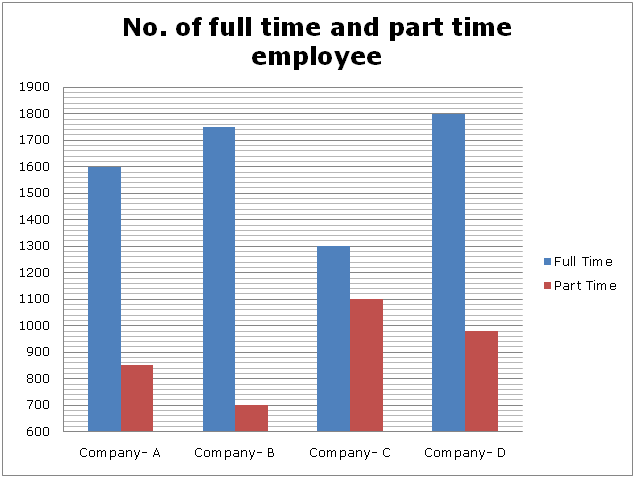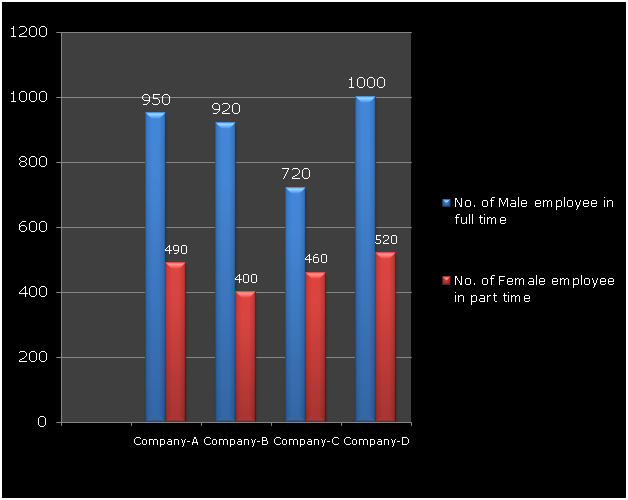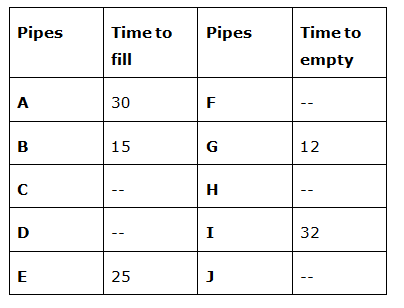# SBI Clerk Mains Quantitative Aptitude (Day-01)

Dear Aspirants, Our IBPS Guide team is providing new series of Quantitative Aptitude Questions for SBI Clerk Mains 2020 so the aspirants can practice it on a daily basis. These questions are framed by our skilled experts after understanding your needs thoroughly. Aspirants can practice these new series questions daily to familiarize with the exact exam pattern and make your preparation effective.

Start Quiz

Ensure Your Ability Before the Exam – Take SBI Clerk 2020 Mains Free Mock Test

Data Interpretation

Directions (1 – 5): Study the following information carefully and answer the questions given below?

In the first bar-chart information about number of total employee of company A, B, C and D who work for part time or full time is given and in the second bar chart information about number of male employee of full time and number of female employee of part time of respective company has been given.1) In how many companies the number of male employees is more than women employees?

a) 1

b) 3

c) 2

d) 4

e) Cannot be determined

2) If the number of men working for part-time increases every year by 10%, what will be the average number of men working for part-time of all companies in  next year?

a) 436

b) 480

c) 484

d) 492

e) 520

3) What is the ratio of the average number of women working for full-time in all companies with the total number of employees in Company A?

a) 143: 490

b) 243: 523

c) 113: 189

d) 137: 248

e) 141: 241

4) All male employees working for the part time in company D, leave the company and move to Company B. What percentage of the number of employees in Company B will be increased?

a) 52.53%

b) 39.63%

c) 18.77%

d) 54.21%

e) 63.53%

5) 25% women of company C who work for part time are now working for full time. Find out what is the percentage of total number of full-time women employee in Company C to the total number of men in company C?

a) 55%

b) 52.33%

c) 59.21%

d) 50.81%

e) 51.10%

Data Interpretation

Directions (6 – 10): Study the following information carefully and answer the questions given below?

The given table shows the time taken (in hours) by different pipes (A, B, C, D and E) to fill and time taken (in hours) by different pipes (F, G, H, I and J) to empty a tank.6) If Pipe A and B started to fill the tank after 5 hours closed and then pipe D and E together can fill the remaining tank in 7(9/13) hours, in how many hours pipe D fill the tank?

a) 30 hours

b) 40 hours

c) 50 hours

d) 20 hours

e) None of these

7) If B and H filled one – tenth of the tank in 6 hours and the efficiency of pipe J to H is 1: 2, in how many hours E and J together can completely fill the tank?

a) 62(2/3) hours

b) 64(2/3) hours

c) 66(2/3) hours

d) 68(2/3) hours

e) None of these

8) Ratio of the efficiency of pipe C and E is 5: 8. If the pipes C and J opened together and after 4.5 hours pipe C is closed and then pipe J and D together can fill the remaining tank, in how many hours required to fill the tank?

a) 12 hours

b) 15 hours

c) 20 hours

d) Cannot be determine

e) None of these

9) If the ratio of time taken by pipes F, H and J alone empties the tank is 6: 5: 10 and the F alone emptied the tank in 24 hours, what is time taken by pipes A and J together to fill the tank?

a) 120 hours

b) 80 hours

c) 100 hours

d) 90 hours

e) None of these

10) What is the ratio of filling capacity of pipe B to emptying of I?

a) 16: 9

b) 16: 7

c) 2: 1

d) 5: 2

e) None of these

Directions (1-5):

Number of male employee in company – A = 950 + 850 – 490 = 1310

Number of female employee in company – A = 1600 + 850 – 1310 = 1140

Number of male employee in company – B = 920 + 700 – 400 = 1220

Number of female employee in company – B = 1750 + 700 – 1220 = 1230

Number of male employee in company – C = 720 + 1100 – 460 = 1360

Number of female employee in company – C = 1300 + 1100 – 1360 = 1040

Number of male employee in company – D = 1000 + 980 – 520 = 1460

Number of female employee in company – D = 1800 + 980 – 1460 = 1320

Total number of male employee working for part-time in next year

= 110% of (850 – 490 + 700 – 400 + 1100 – 460 + 980 – 520)

= 1760 * 110/100

= 1936

Required Average = 1936/4 = 484

Number of women working for full-time in all companies

= (1600 – 950 + 1750 – 920 +1300 – 720 + 1800 – 1000) = 2860

Average of women working for full-time in all companies

= 2860/4 = 715

And total no of employees in company A = 1600 + 850 = 2450

Required Ratio = 715: 2450 = 143: 490

Male employee of company D who working for part time = 980 – 520 = 460

Total number of employee in company B = 2450

After D join B = 2450 + 460 = 2910

% increased in company B = (460/2450) x 100 = 18.77%

25% women of company –c who work for part time = 460 * 25% = 115

Now total woman work in full time in company – C = 115 + 580 = 695

Required percentage = (695/1360) x 100

= 51.10%

Directions (6-10) :

Pipes A and B together can fill the tank in 5 hours = (1/30 + 1/15) * 5

= 5/10 = ½

Remaining part = 1 – ½ = ½

C and D together can fill ½ of the tank in 7(9/13) hours

(1/25 + 1/x) * 100/13 = ½

1/25 + 1/x = 13/200

1/x = 5/200

1/x = 1/40

x = 40 h

(1/15 – 1/H) * 6 = 1/10

1/H = 1/15 – 1/60

1/H = 1/20

Time ratio of J and H = 2: 1

Pipe J emptied the tank in = 2/1 * 20 = 40 hours

E and J together can fill the tank = 1/25 – 1/40

= 15/1000

Required time = 200/3 hours = 66(2/3) hours

Time ratio of Pipe C and E = 8: 5

C alone fill the tank = 8/5 * 25 = 40

H alone empties the tank = 5/6 * 24 = 20 hours

J alone empties the tank = 10/6 * 24 = 40 hours

A + J together can fill the tank = 1/30 – 1/40 = 1/120